Question

# A password consists of one letter followed by a five-digit number. A) How many passwords are...

A password consists of one letter followed by a five-digit number.

A) How many passwords are possible if none of the letters or digits can be repeated?

B) What is the probability of guessing the password in one trial if there are no restrictions? (To find the probability use the definition of classical probability.)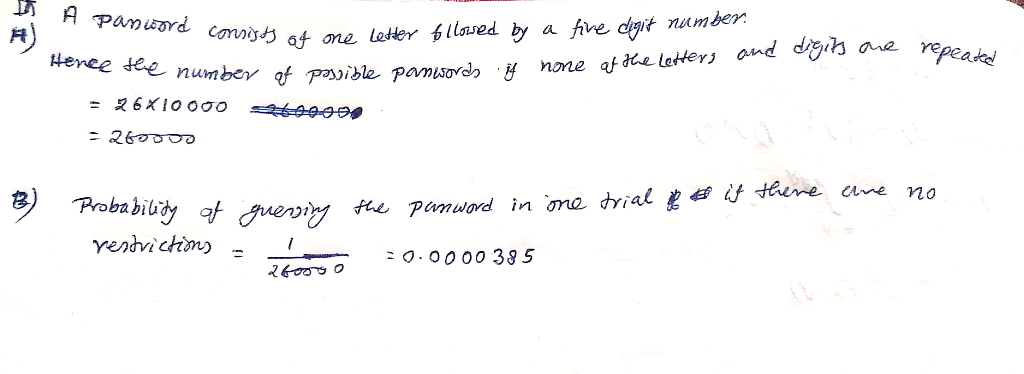#### Earn Coins

Coins can be redeemed for fabulous gifts.

Similar Homework Help Questions
• ### A password consists of three letters followed by a two-digit number. (a) How many passwords are...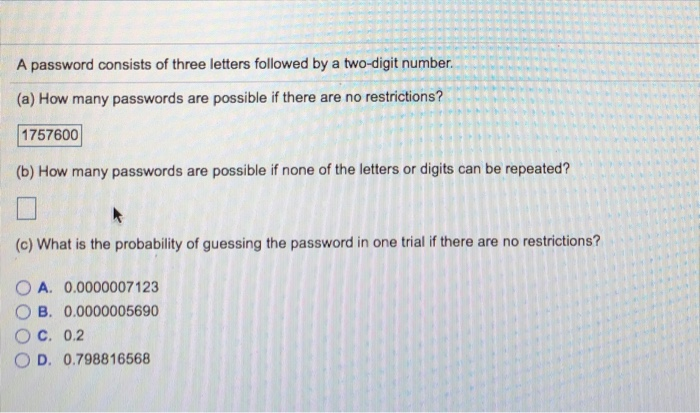A password consists of three letters followed by a two-digit number. (a) How many passwords are possible if there are no restrictions? 1757600 (b) How many passwords are possible if none of the letters or digits can be repeated? (c) What is the probability of guessing the password in one trial if there are no restrictions? O A. 0.0000007123 O B. 0.0000005690 O C. 0.2 O D. 0.798816568

A 5-character password must start with a letter followed by 4 digits. How many passwords are possible? (Digits and letters may be repeated).

• ### A password to a computer consists of 6 characters: a digit, a letter, a digit, a...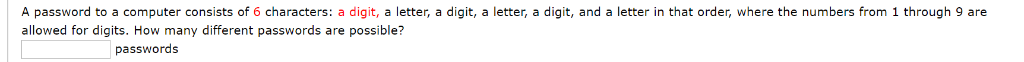A password to a computer consists of 6 characters: a digit, a letter, a digit, a letter, a digit, and a letter in that order, where the numbers from 1 through 9 are allowed for digits. How many different passwords are possible? passwords

• ### A code word consists of 2 letters followed by 7 digits. The first letter must be...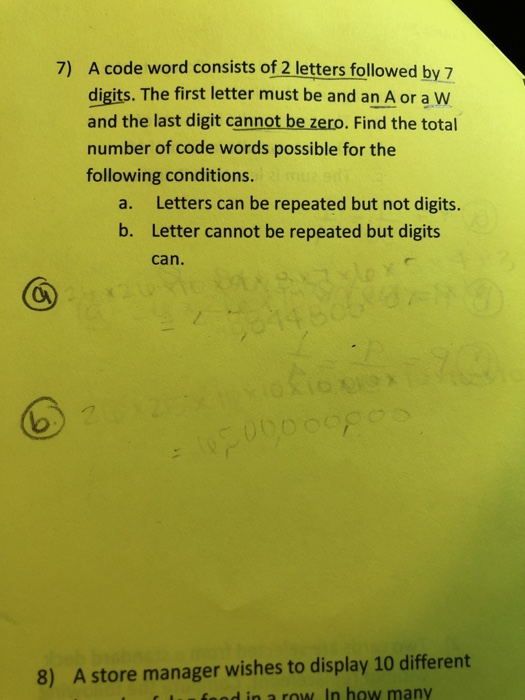A code word consists of 2 letters followed by 7 digits. The first letter must be and an A or a w and the last digit cannot be zero. Find the total number of code words possible for the following conditions. 7) Letters can be repeated but not digits Letter cannot be repeated but digits can a. b. 8) A store manager wishes to display 10 different f food in a row In how many

• ### A computer password is required to be 9 characters long. How many passwords are possible if...

A computer password is required to be 9 characters long. How many passwords are possible if the password requires 2 letter(s) followed by 7 digits (numbers 0-9), where no repetition of any letter or digit is allowed? There are _________ possible passswords.

• ### if a license plate consists of 2 letters followed by 4 digits, how many different plates could be created having at least one letter or digit repeated

if a license plate consists of 2 letters followed by 4 digits, how many different plates could be created having at least one letter or digit repeated? I know its not 6,760,000

• ### The serial number on a dollar bill consists of a letter, followed by eight digits and...

The serial number on a dollar bill consists of a letter, followed by eight digits and then a letter. How many different serial numbers are possible if letters and digits cannot be repeated?

• ### Suppose you have to create a password consisting of seven letters followed by two digits. The...

Suppose you have to create a password consisting of seven letters followed by two digits. The letters cannot be repeated but the digits can be repeated. a) How many possible such passwords can you create? b) If you insist on alternating consonants with vowels (do not count “y” as a vowel), starting and ending the string of letters with consonants, and making the two digits at the end a prime number greater than 23, then how many passwords can you...

• ### A password consists of 4 letters among 26 lower-case English alphabet letters and 10 digits: 0,1,...,9....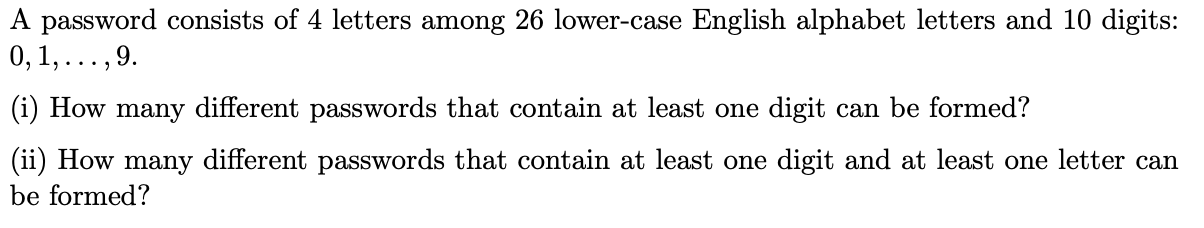A password consists of 4 letters among 26 lower-case English alphabet letters and 10 digits: 0,1,...,9. (i) How many different passwords that contain at least one digit can be formed? (ii) How many different passwords that contain at least one digit and at least one letter can be formed?

• ### A password must begin with two letters followed by four digits. What is the number of...

A password must begin with two letters followed by four digits. What is the number of possible passwords of this type if characters may be repeated?